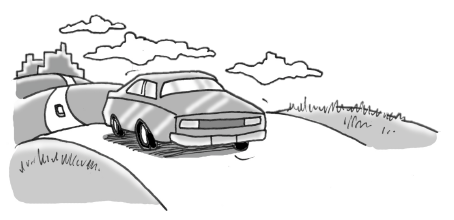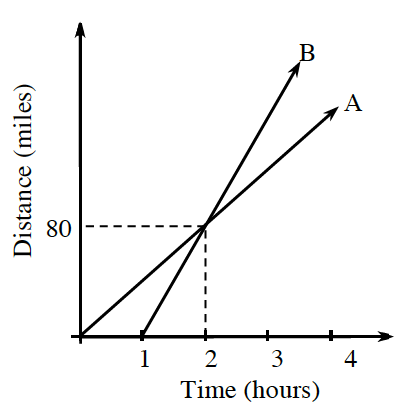### Home > AC > Chapter 3 > Lesson 3.1.1 > Problem3-8

3-8.

GO GOLDEN GOPHERS!

The graph below describes the distance two cars have traveled after leaving a football game at the University of Minnesota.1. Which car was traveling faster? How can you tell?

You can find this by discovering which car traveled a greater distance in a shorter amount of time.

Car B was traveling faster.

Its line is steeper.

2. The lines cross at $(2,80)$. What does this point represent?

What are the units for the $x$ and $y$ coordinates?

In $2$ hours, both cars will be $80$ miles from the game.

3. Assuming that Car A continued to travel at a constant rate, how far did Car A travel in the first $4$ hours?

Because Car A traveled $80$ miles in $2$ hours at a constant rate, it went $40$ miles in $1$ hour.
If the Car A travels $40$ miles every hour, how far will it travel in $4$ hours?